# Quiz 8: Project Management

Business

Company HMC negotiates a deal with Company CR to build six houses for the development of the town. The activity, predecessor, and times estimates of the project are shown in the following table: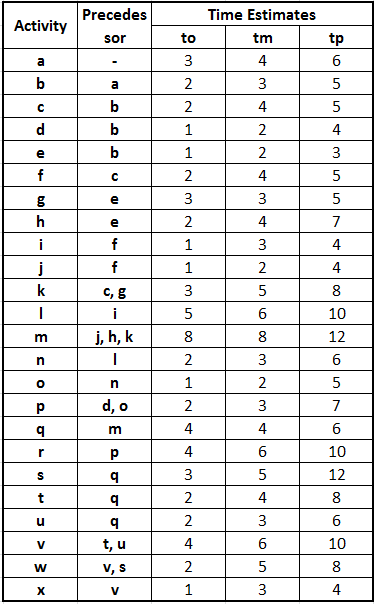Table 1 Prepare the project network with the help of predecessor relationship as shown below: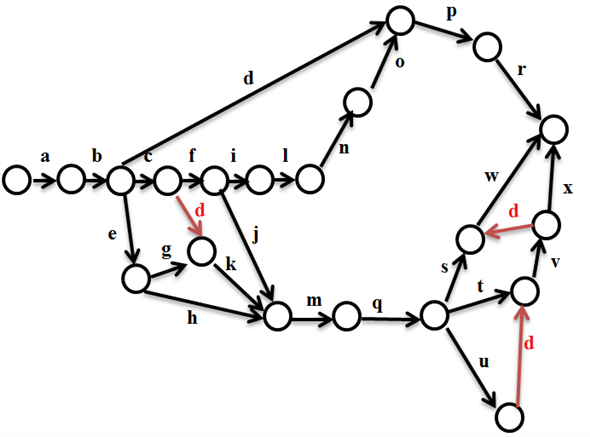Figure 1 Calculate the expected time, variance, and standard deviation for each activity using the following formulas: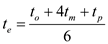…… (1)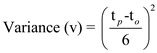…… (2)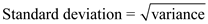…… (3) Where,Prepare the excel spreadsheet and calculate expected time and total time for each path as shown below: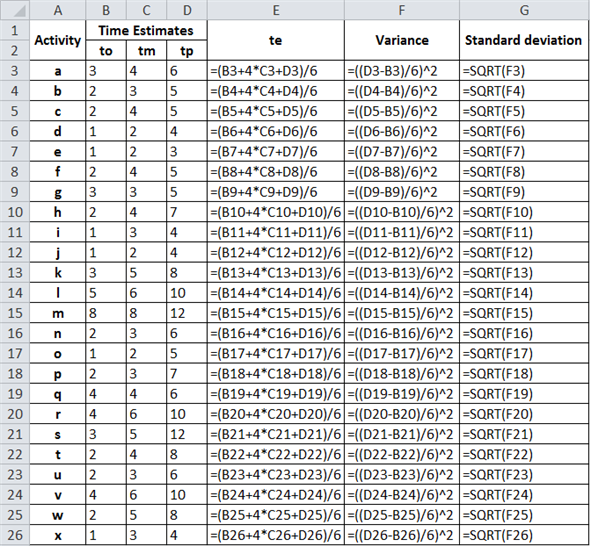Table 2 The following results are obtained :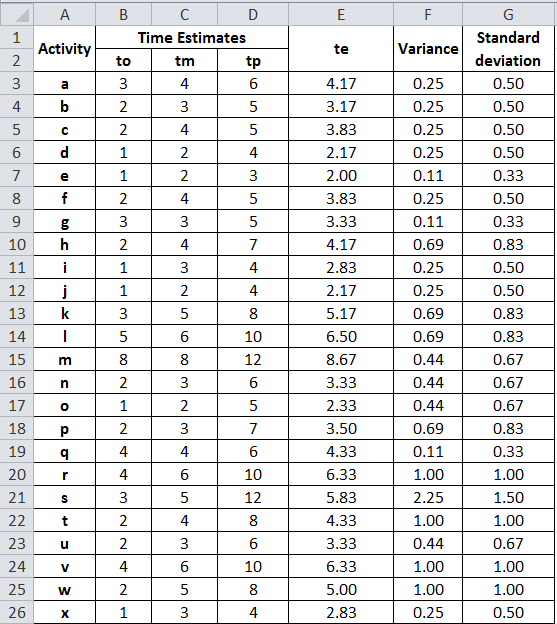Table 3 Calculate ES, LS, EF, LF, and Slack using the following formulas: ES = latest EF for all immediate predecessors …… (4) EF = ES + activity's duration …… (5) LF = earliest LS for all immediate predecessors …… (6) LS = LF - activity's duration …… (7) Where, ES = Earliest start time for a project activity EF = Earliest finish time for a project activity LF = Latest finish time for a project activity LS = Latest start time for a project activity Prepare the table and calculate the values of ES, LS, EF, LF, and Slack as shown below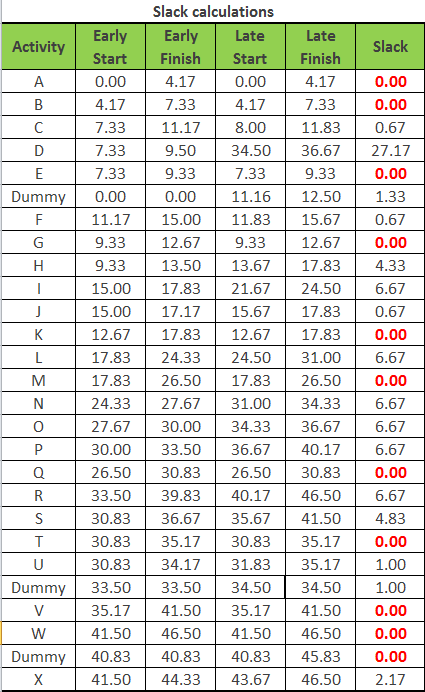Table 4 From the above table, the path having zero slack is considered to be as critical path. Therefore, Critical path would be a-b-e-g-k-m-q-t-v-dummy-w. Calculate the duration of the path as shown below: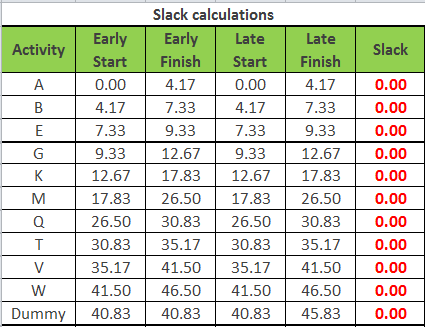Table 5 Thus, the project duration is 45.8 days for the project to be complete under the critical path. Calculate the Z value for the project to be completed on or before 45 days using the following formula: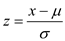…… (2) Substitute the values in equation (2)Calculate the probability using excel spreadsheet, as shown below: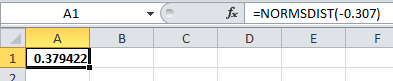Table 6 Thus, the Probability that the concert preparation would be completed in 45 days is 0.379 (b) Company HMC should move its resources from the activities that have slack towards the critical activities. Similarly, activities such as d and e having slack can be moved to k and m which are critical.

First to solve this problem, use the QM for Window software (provided with the book) following these instructions: First, open the software; under module, select Project Management. Next, open a blank document and select "Single Time Estimate". A menu will open; enter the number of activities which is 4 for this problem (make sure you have selected numbers for listing). Select OK and a window will show in order to enter the information. Enter the values; then, select solve on the top right corner and a series of windows with solutions will open. The window titled: Project Management has the solution and Charts has the graph.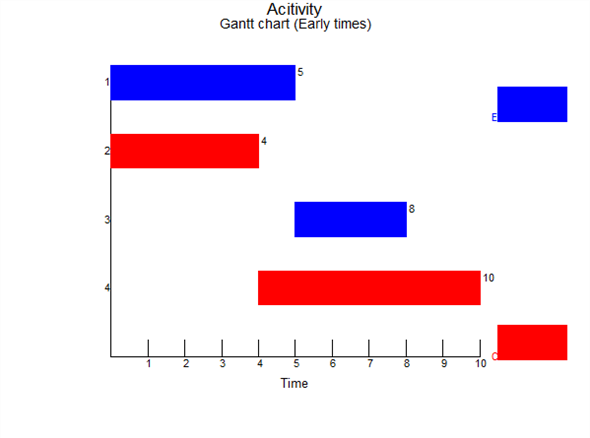The completion time is 1-3-4 for a total of 10

Step 1: Calculate the mean expected time and variance of the concert project scheduling as per the formula, shown below:Here, a= optimistic time estimate m=most likely time estimate b=pessimistic time estimate Calculate the mean time and variance for the 1 st activity as: t = 2+4*4+7/ 6 = 4.16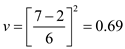Similarly, the mean time and variance for each activity is shown as follows: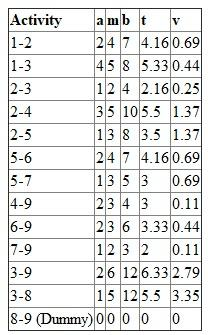Step2: Develop a network diagram for the schedule and mean time taken to complete each activity, as shown below: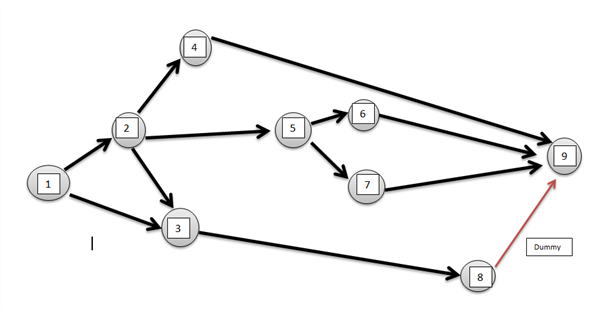Obtain the Earliest start (ES), Earliest Finish (EF), Latest Start (LS) and Latest Finish (LF) with the help of the network diagram and the mean time of every activity, as shown below: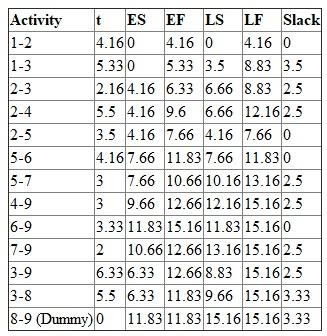From the above table, slack of each activity is given by: ES - LS. Step 3: Obtain the critical path of the activity so as to obtain the duration of the path, as shown below: Critical path has zero slack and as per the table, the activities corresponding to zero slack are: 1-2-5-6-9 The duration of the path is calculated as follows: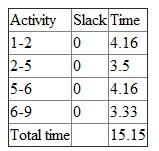Thus, the project duration is 15.15 days for the project to be complete under the critical path. Step 4: Calculate the probability for the project to be completed on or before 18 days, is shown as follows: Obtain the standard deviation of the path by using the variance: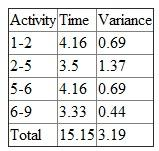Standard deviation = vvariance =v3.19 = 1.78 Thus, the P(x = 18)From the normal table the value of Z for 1.58 is.4429 and the probability is given by 0.5+0.4429= 0.942 Thus, there is 94% probability that the concert preparation will be completed in 18 days.